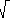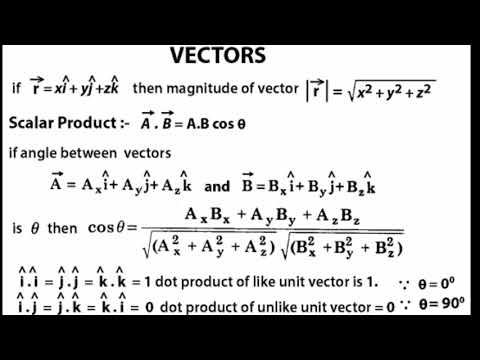×#### Thank you for registering.

One of our academic counsellors will contact you within 1 working day.

Click to Chat

1800-1023-196

+91-120-4616500

CART 0

• 0

MY CART (5)

Use Coupon: CART20 and get 20% off on all online Study Material

ITEM
DETAILS
MRP
DISCOUNT
FINAL PRICE
Total Price: Rs.

There are no items in this cart.
Continue Shopping

all (A to Z) formula of vector for class 11


8 years ago

							Dear Shubham,
The formula for the length of a 2D vector is the Pythagorean Formula. Say that the vector is represented by   (x, y)T.   Put the vector with its tail at the origin. Now make a triangle by drawing the two sides:
side_1   =  (x, 0)T

side_2   = (0, y)T.

The length of side_1 is x, and the length of side_2 is y, so:

length (x, y)T   =( x2 + y2 )

In this formula,means the positive square root. We don''t (of course) want the length to be negative.
Cracking IIT just got more exciting,It s not just all about getting assistance from IITians, alongside Target Achievement and Rewards play an important role. ASKIITIANS has it all for you, wherein you get assistance only from IITians for your preparation and win by answering queries in the discussion forums. Reward points 5 + 15 for all those who upload their pic and download the ASKIITIANS Toolbar, just a simple  to download the toolbar….
So start the brain storming…. become a leader with Elite Expert League ASKIITIANS
Thanks
Aman Bansal


8 years ago
							Dear Shubham,
vector quantity has both magnitude and direction. Acceleration, velocity, force and displacement are all examples of vector quantities. A scalar quantity is has only magnitude (so the direction is not important). Examples include speed, time and distance.
Unit Vectors
A unit vector is a vector which has a magnitude of 1. There are three important unit vectors which are commonly used and these are the vectors in the direction of the x, y and z-axes. The unit vector in the direction of the x-axis is i, the unit vector in the direction of the y-axis is j and the unit vector in the direction of the z-axis is k.
Writing vectors in this form can make working with vectors easier.
The Magnitude of a Vector
The magnitude of a vector can be found using Pythagoras''s theorem.

The magnitude of ai + bj = √(a2 + b2)

We denote the magnitude of the vector a by | a |
Position Vectors
Position vectors are vectors giving the position of a point, relative to a fixed point (the origin).
For example, the points A, B and C are the vertices of a triangle, with position vectors a, b and c respectively:You can draw in the origin wherever you want.
Notice that= - a + b = b - a because you can get from A to B by going from A to O and then going from O to B.
Cracking IIT just got more exciting,It s not just all about getting assistance from IITians, alongside Target Achievement and Rewards play an important role. ASKIITIANS has it all for you, wherein you get assistance only from IITians for your preparation and win by answering queries in the discussion forums. Reward points 5 + 15 for all those who upload their pic and download the ASKIITIANS Toolbar, just a simple  to download the toolbar….
So start the brain storming…. become a leader with Elite Expert League ASKIITIANS
Thanks
Aman Bansal


8 years ago
							Vector FormulasA vector can also be defined as an element of a vector space.  Vectors are sometimes referred to by the number of coordinates they have, so a 2-dimensional vector  is often called a two-vector, an n-dimensional vector is often called an n-vector, and so on.The important formulas of vectors are given below: 1. The position vector of any point p(x,y) is$op = \dbinom{x}{y}$ or OP = ( x,y ). 2.The magnitude of position vector$OP = \sqrt{x^2 + y^2}$ and direction$\tan \theta = \dfrac{y}{x}$ 3. The unit vector =$\dbinom{1}{0}$ where the magnitude of unit vector is 1Or,the unit vector =$\dfrac{vector}{its modulus} = \dfrac{ \overrightarrow{a}}{ |\overrightarrow{a}| }$ 4.The two vectors$\overrightarrow{a}$ and$\overrightarrow{b}$ are parallel if$\overrightarrow{a} = k \overrightarrow{b}$ and$\overrightarrow{b} = m \overrightarrow{a}$ where k and m are the scalars. 5.If$\overrightarrow{AB} + \overrightarrow{BC} = \overrightarrow{AC}$ then$\overrightarrow{AC}$ is the result vector which is the triangle law of vector addition. 6. The scalar or dot product of any two vectors$\overrightarrow{a} . \overrightarrow{b} = | \overrightarrow{a} | | \overrightarrow{b} | cos\theta$. 7. The angle between two vectors is$\cos \theta = \dfrac{\overrightarrow{a} . \overrightarrow{b}} {| \overrightarrow{a} | | \overrightarrow{b} |}$ 8.$\overrightarrow{a} = x_1 i + y_1 j$ and$\overrightarrow{b} = x_2 i + y_2 j$ , then :$\overrightarrow{a} . \overrightarrow{b} = x_1 . x_2 + y_1 . y_2$ where$i.j = j.1 = 0$ 9. If the position vector of A is$\overrightarrow{a}$ , position vector of point B is$\overrightarrow{b}$ and position vector of mid-point M is m then$\overrightarrow{m} = \dfrac{\overrightarrow{a} + \overrightarrow{b}}{2}$ 10. If the point P divides Ab internally in the ratio m:n then position vector of P is given by$\overrightarrow{p} = \dfrac{n \overrightarrow{a} + m \overrightarrow{b}}{m + n}$ which is a section formula. 11.If P divides AB externally in the ratio m:n then$\overrightarrow{p} = \dfrac{m \overrightarrow{b} - n \overrightarrow{a}}{m - n}$PRODUCT OF TWO VECTORS 1.Scalar Product ( dot product ) Let$\overrightarrow{a} = (a_1,a_2) , \overrightarrow{b} = (b_1,b_2)$ then dot product of$\overrightarrow{a}$ &$\overrightarrow{b}$ is devoted by$\overrightarrow{a}.\overrightarrow{b}$ read as$\overrightarrow{a}$ dot$\overrightarrow{b}$ and defined by$\overrightarrow{a}.\overrightarrow{b} = a_1 b_1 , a_2 b_2$ Note:if$\overrightarrow{a} = (a_1,a_2,a_3),\overrightarrow{b} = (b_1,b_2,b_3) \\ \overrightarrow{a} . \overrightarrow{b} = a_1b_1 + a_2b_2 +a_3b_3$ OR The scalar product of$\overrightarrow{a}$ &$\overrightarrow{b}$ is devoted by$\overrightarrow{a} . \overrightarrow{b}$ ,$\overrightarrow{a} . \overrightarrow{b} |\overrightarrow{a}|.|\overrightarrow{b}| \cos \theta$ where$\theta$ being  angle between$\overrightarrow{a}$ &$\overrightarrow{b}$ Note:1$\cos \theta = \dfrac{\overrightarrow{a} . \overrightarrow{b}}{| \overrightarrow{a} | | \overrightarrow{b} |}$Note:2$\overrightarrow{a}$ &$\overrightarrow{b}$ are perpendicular if$\theta$ =$90^o$i.e$\overrightarrow{a} . \overrightarrow{b} |\overrightarrow{a}|.|\overrightarrow{b}| \cos 90^o$ or$\overrightarrow{a} . \overrightarrow{b} = 0$ 2.Properties of Scalar Producti.$\overrightarrow{a} . \overrightarrow{b} = \overrightarrow{b} . \overrightarrow{a}$.ii.$m \overrightarrow{a} . n \overrightarrow{b} - mn \overrightarrow{a}. \overrightarrow{b} = \overrightarrow{a} mn \overrightarrow{b}$.iii.$\overrightarrow{a}( \overrightarrow{b} + \overrightarrow{c} ) = \overrightarrow{a} . \overrightarrow{c}$iv.$( \overrightarrow{b} + \overrightarrow{c} )^2 = \overrightarrow{a}^2 + 2.\overrightarrow{a}.\overrightarrow{b} + \overrightarrow{b}^2$v. If$\overrightarrow{i} = (1,0,0): \overrightarrow{j} = (0,1,0), \overrightarrow{k} = (0,0,1)$then$\overrightarrow{i} . \overrightarrow{j} = \overrightarrow{j} . \overrightarrow{k} = \overrightarrow{k} . \overrightarrow{i} = \overrightarrow{i} . \overrightarrow{k} = \overrightarrow{j} . \overrightarrow{k} = 0$ 3.Vector (cross) Product of two vectors.Let$\overrightarrow{a} = (a_1 , a_2 , a_3 ), \overrightarrow{b} = (b_1 , b_2 , b_3 )$ be two vectors then the cross product of$\overrightarrow{a} \times \overrightarrow{b}$is devoted by$\overrightarrow{a} \times \overrightarrow{b}$ and defined by$\overrightarrow{a} \times \overrightarrow{b} = (a_1 , a_2 , a_3 ) \times (b_1 , b_2 , b_3 )$$= \begin{pmatrix} a_1 & a_2 & a_3 & a_1 & a_2 \\ b_1 & b_2 & b_3 & b_1 & b_2 \end{pmatrix}$ =$( a_2 b_3 - a_3 b_2 , a_3 b_1 - a_1 b_2 - a_2 b_1 )$ We can define in terms of determinants as follows$\overrightarrow{a} \times \overrightarrow{b}$ =$\begin{vmatrix} \overrightarrow{i} & \overrightarrow{j} & \overrightarrow{k} \\ a_1 & a_2 & a_3 \\ b_1 & b_2 & b_3 \end{vmatrix}$$= ( a_2 b_3 - a_3 b_2 ) \overrightarrow{i} + ( a_3 b_1 - a_1 b_3 ) \overrightarrow{j} + ( a_1 b_2 - a_2 b_1 ) \overrightarrow{k}$Note:1$| \overrightarrow{a} \times \overrightarrow{b} | = | \overrightarrow{a} | \times | \overrightarrow{b} | \sin \theta , \theta$ being angle between$\overrightarrow{a}$ &$\overrightarrow{b}$Note:2$\dfrac{| \overrightarrow{a} \times \overrightarrow{b}| }{| \overrightarrow{a} | | \overrightarrow{b} | \sin \theta }$Note:3               If$\theta = 0$ , the$| \overrightarrow{a} \times \overrightarrow{b} | = 0 i.e \overrightarrow{a} \times \overrightarrow{b} = 0$ and$\overrightarrow{a}$ &$\overrightarrow{b}$ are parallel if$\overrightarrow{a} \times \overrightarrow{b} = 0$. 4. Properties of cross product i.$\overrightarrow{a} \times \overrightarrow{b} = 0$ii.$\overrightarrow{a} \times \overrightarrow{b} = \overrightarrow{b} \times \overrightarrow{a}$iii.$\overrightarrow{a} \times ( \overrightarrow{b} + \overrightarrow{c} ) = \overrightarrow{a} \times \overrightarrow{b} + \overrightarrow{a} \times \overrightarrow{c}$iv.$( n \overrightarrow{a} ) \times \overrightarrow{b} = n( \overrightarrow{a} \times \overrightarrow{b} ) \times n \overrightarrow{b}$v.$\overrightarrow{a} \times \overrightarrow{b}$ is perpendicular to both$\overrightarrow{a}$ and$\overrightarrow{b}$vi.$| \overrightarrow{a} \times \overrightarrow{b} |$ is a Area of paralelogram with sides$\overrightarrow{a}$ and$\overrightarrow{b}$vii.$\dfrac{1}{2} | \overrightarrow{a} \times \overrightarrow{b} + \overrightarrow{b} \times \overrightarrow{c} + \overrightarrow{c} \times \overrightarrow{a} |$ = area of triangle having$\overrightarrow{a}$ ,$\overrightarrow{b}$ ,$\overrightarrow{c}$ as position vectors of vertices of a triangle.

3 years ago
							hello studentshope it helpsthankyou

4 months ago
Think You Can Provide A Better Answer ?

## Other Related Questions on Vectors

View all Questions »### Course Features

• 731 Video Lectures
• Revision Notes
• Previous Year Papers
• Mind Map
• Study Planner
• NCERT Solutions
• Discussion Forum
• Test paper with Video Solution

### Course Features

• 19 Video Lectures
• Revision Notes
• Test paper with Video Solution
• Mind Map
• Study Planner
• NCERT Solutions
• Discussion Forum
• Previous Year Exam Questions